# Graph of Polynomial

A Polynomial function is an algebraic expressions that satisfied quadratic function, cubic function format ...etc

## Example 1

The diagram below shows the graph of $Y ={x}^{3}+{x}^{2}-5x+3$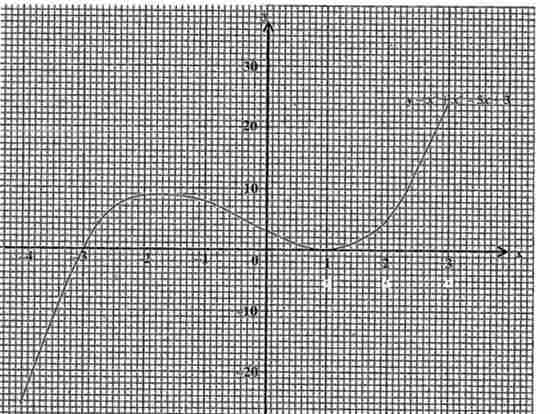Use the graph

(i) to calculate an estimate of the gradient of the curve at the point (2,5)

(ii) to solve the equations

(a) ${x}^{3}+{x}^{2}-5x+3= 0$

(b) ${x}^{3}+{x}^{2}-5x+3= 5x$

(iii) to calculate an estimate of the area bounded by the curve, x = 0, y = 0 and x = -2

Solutions

(i)

Use the graph to find gradient

(2, 5) (2.5, 10)

Gradient = $\frac{\mathrm{Y2 - Y1}}{X2 - X1}$

X1 = 2, X2 = 2.5, Y1 = 5, Y2 = 10

Gradient = $\frac{\mathrm{10 - 5}}{2.5 - 2}$

Gradient = $\frac{5}{0.5}$

Answer: Gradient = $10$

(ii)

(a) ${x}^{3}+{x}^{2}-5x+3= 0$

From the equation above $Y ={x}^{3}+{x}^{2}-5x+3$.

Therefore $y= 0$

Draw a line $y= 0$ on the graph. hence find the values of x at the points the line intersect the graph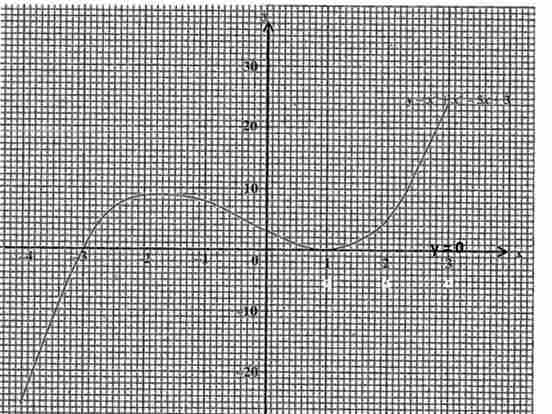values of x at the points the line $y= 0$ touchs the graph

Answer: (1, 0) and (-3, 0) from line $y= 0$

(b) ${x}^{3}+{x}^{2}-5x+3= 5x$

$y ={x}^{3}+{x}^{2}-5x+3$

$y= 5x$

Plot the line $y= 5x$ on the graph

given that x = -3 , y = 5x (-3, -15) and x = 3, y = 5x (3, 15)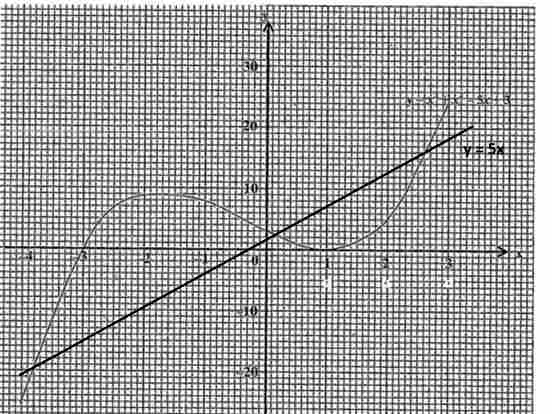values of x at the points the line $y= 5x$ touchs the graph

Answer: (-4, -20) and (0.2, 0.2) and (2.5, 16)

(iii)

Area bounded by lines x = 0, y = 0 and x = -2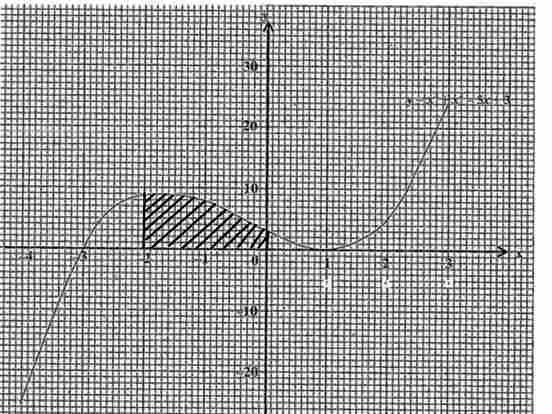The shape of the area bounded by lines x = 0, y = 0 and x = -2 is a tripezium

##### Formula for area of a tripezium

Area = $\frac{\mathrm{a + b}}{2}$ h

a = 2, b = 1, h = 9

Area = $\frac{\mathrm{2 + 1}}{2}$ 9

Area = $\frac{3}{2}$ 9

Area = $\frac{\mathrm{27}}{2}$

Answer: Area = $13.5{\mathrm{units}}^{2}$

## Example 2

The variables x and y are connected by the equation $Y =3-5x+{x}^{2}+{x}^{3}$. some of the corresponding values of x and y are given in the table below

$\begin{array}{|llllllll|}\hline x& -3& -2& -1& 0& 1& 2& 3\\ y& 0& 9& r& 3& 0& 5& 24\\ \hline\end{array}$

i) Calculate Value of r

ii) Taking 2cm to represent 1 unit on the x-axis for -3 =< x =< 3 and 2cm to represent 10 units on the y-axis for 0 =< y =< 30, draw the graph of $Y =3-5x+{x}^{2}+{x}^{3}$

iii) Use your graph to calculate an estimate of the

a) gradient of the curve at the point where x = 2,

b) area bounded by the curve, x = -3 and x = -2.

Solution:

i)

$Y =3-5x+{x}^{2}+{x}^{3}$

Y = r

x = -1

$r =3-5\left(-1\right)+{\mathrm{\left(-1\right)}}^{2}+{\mathrm{\left(-1\right)}}^{3}$

$r =3+5+1+\mathrm{\left(-1\right)}$

$r =3+5+1-1$

Answer: $r =8$

(ii)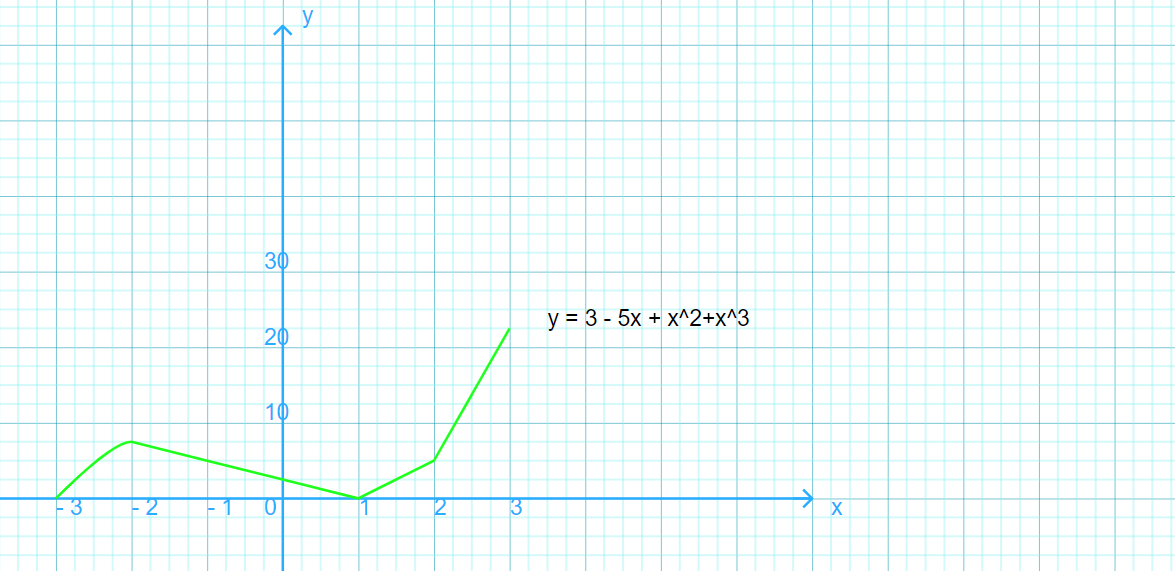(iii) (a) and (b) using the gragh

(a)

Gradient = $\frac{\mathrm{Y2 - Y1}}{X2 - X1}$

(2, 5), (3, 15)

X1 = 2, X2 = 3, Y1 = 5, Y2 = 15

Gradient = $\frac{\mathrm{15 - 5}}{3 - 2}$

Gradient = $\frac{\mathrm{10}}{1}$

Answer: Gradient = $\mathrm{10}$

(b)

The shape of the area bounded by line x = -3 and x = -2 is a triangle, hence to find the area you have to use the formula for area of a triangle

##### Formula for area of a triange

Area = $\frac{1}{2}b×h$

b = 1 , h = 9

Area = $\frac{1}{2}1×9$

Area = $\frac{9}{2}$

Answer: Area = ${\mathrm{4.5 units}}^{2}$